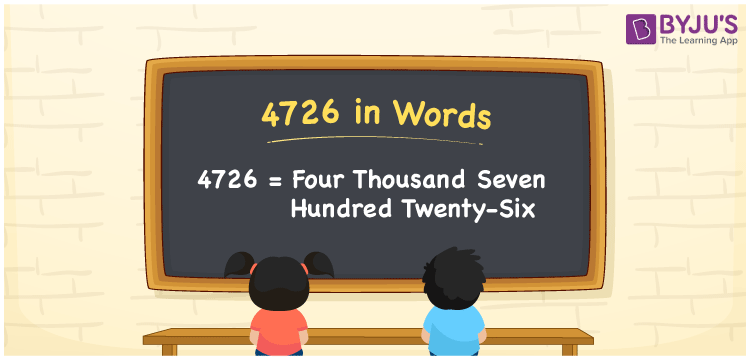# 4726 in Words

4726 in words is Four thousand seven hundred twenty-six. We know that 4726 is a cardinal number since it denotes a specific quantity. Suppose you paid Rs. 4726 for a new sofa, then you can say, “I spent Four thousand seven hundred twenty-six rupees for a new sofa”. Here, 4726 represents the price of the sofa, and Four thousand seven hundred twenty-six is the word form of the cardinal number 4726.

 4726 in words Four thousand seven hundred twenty-six Four thousand seven hundred twenty-six in Numbers 4726

## 4726 in English Words

We generally write numbers in words with the help of the English alphabet. So, we can read 4726 in English as “Four thousand seven hundred twenty-six”.## How to Write 4726 in Words?

The below table depicts the place value chart with four columns since the number 4726 contains four digits.

 Thousands Hundreds Tens Ones 4 7 2 6

Here, ones = 6, tens = 2, hundreds = 7, and thousands = 4.

By expanding these numbers with the respective place values, we get;

4 × Thousand + 7 × Hundred + 2 × Ten + 6 × One

= 4 × 1000 + 7 × 100 + 2 × 10 + 6 × 1

= 4000 + 700 + 20 + 6

= Four thousand + Seven hundred + Twenty + Six

= Four thousand seven hundred twenty-six

Therefore, 4726 in words = Four thousand seven hundred twenty-six.

4726 is a natural number that precedes 4727 and succeeds 4725.

4726 in words – Four thousand seven hundred twenty-six

Is 4726 an odd number? – No

Is 4726 an even number? – Yes

Is 4726 a prime number? – No

Is 4726 a composite number? – Yes

Is 4726 a perfect square number? – No

Is 4726 a perfect cube number? – No

## Frequently Asked Questions on 4726 in Words

Q1

### Write the number 4726 in words.

We can write the number 4726 in words as Four thousand seven hundred twenty-six.
Q2

### How to write Rs. 4726 in words on a cheque?

On a cheque, Rs. 4726 can be written in words as “Four thousand seven hundred twenty-six rupees only”.
Q3

### Write the value 4726 – 3108 in words.

4726 – 3108 = 1618 Hence, the value of 4726 – 3108, i.e., 1618 in written in words as One thousand six hundred and eighteen.